# The Azimuth Project Blog - networks of dynamical systems

This page is a blog article in progress, written by Eugene Lerman. To see discussions of this article while it is being written, visit the Azimuth Forum.

guest post by Eugene Lerman

Hi, I’m Eugene Lerman. I met John back in the mid 1980s when John and I were grad students at MIT. John was doing mathematical physics and I was studying symplectic geometry. We never talked about networks. Now I teach in the math department at the University of Illinois at Urbana, and we occasionally talk about networks on his blog.

A few years ago a friend of mine who studies locomotion in humans and other primates asked me if I knew of any math that could be useful to him.

I remember coming across an expository paper on ‘coupled cell networks’:

• Martin Golubitsky and Ian Stewart Nonlinear dynamics of networks: the groupoid formalism, Bull. Amer. Math. Soc. 43 (2006), 305–364.

In this paper, Golubitsky and Stewart used the study of animal gaits and models for the hypothetical neural networks called ‘central pattern generators’ that give rise these gaits as motivation for the study of networks of ordinary differential equations with a strange kind of symmetry. In particular they were interested in ‘synchrony’. When a horse trots, or canters, or gallops, its limbs move in a synchronized way—how does this work?They explained that synchrony could arise when the differential equations have no group symmetries but are ‘groupoid invariant’. I thought that it would be fun to understand what ‘groupoid invariant’ meant and why such invariance leads to synchrony.

I talked my colleague Lee DeVille into joining me on this adventure. Lee had just arrived at Urbana after a postdoc at NYU. After a few years of thinking about these networks Lee DeVille and I realized that strictly speaking one doesn’t really need groupoids for these synchrony results and it’s better to think of the social life of networks instead. Here is what we figured out—a full and much too precise story is here:

Let’s start with an example of a class of ODEs with a mysterious property:

Example. Consider this ordinary differential equation for a function $\vec{x} : \mathbb{R} \to {\mathbb{R}}^3$

$\begin{array}{rcl} \dot{x}_1&=& f(x_1,x_2)\\ \dot{x}_2&=& f(x_2,x_1)\\ \dot{x}_3&=& f(x_3, x_2) \end{array}$

for some function $f:{\mathbb{R}}^2 \to {\mathbb{R}}.$ It is easy to see that a function $x(t)$ solving

$\displaystyle{ \dot{x} = f(x,x) }$

gives a solution of these equations if we set

$\vec{x}(t) = (x(t),x(t),x(t))$

You can think of the differential equations in this example as describing the dynamics of a complex system built out of three interacting subsystems. Then any solution of the form

$\vec{x}(t) = (x(t),x(t),x(t))$

may be thought of as a synchronization: the three subsystems are evolving in lockstep.

One can also view the result geometrically: the diagonal

$\displaystyle{ \Delta = \{(x_1,x_2, x_3)\in {\mathbb{R}}^3 \mid x_1 =x_2 = x_3\} }$

is an invariant subsystem of the continuous-time dynamical system defined by the differential equations. Remarkably enough, such a subsystem exists for any choice of a function $f$.

Where does such a synchronization or invariant subsystem come from? There is no apparent symmetry of ${\mathbb{R}}^3$ that preserves the differential equations and fixes the diagonal $\Delta,$ and thus could account for this invariant subsystem. It turns out that what matters is the structure of the mutual dependencies of the three subsystems making up the big system. That is, the evolution of $x_1$ depends only on $x_1$ and $x_2,$ the evolution of $x_2$ depends only on $x_2$ and $x_3,$ and the evolution of $x_3$ depends only on $x_3$ and $x_2.$

These dependencies can be conveniently pictured as a directed graph: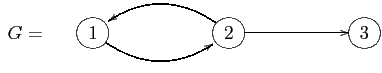The graph $G$ has no symmetries. Nonetheless, the existence of the invariant subsystem living on the diagonal $\Delta$ can be deduced from certain properties of the graph $G/$ The key is the existence of a surjective map of graphs

$\varphi :G\to G'$

from our graph $G$ to a graph $G'$ with exactly one node, call it $a,$ and one arrow. It is also crucial that $\varphi$ has the following lifting property: there is a unique way to lift the one and only arrow of $G'$ to an arrow of $G$ once we specify the target node of the lift.

We now formally define the notion of a network of regions and of a continuous-time dynamical system on such a network. Equivalently, we define a network of continuous-time dynamical systems. We start with a directed graph

$G=\{G_1\rightrightarrows G_0\}$

Here $G_1$ is the set of edges, $G_0$ is the set of nodes, and the two arrows assign to an edge its source and target, respectively. To each node we attach a region (more formally a manifold, possibly with corners). Here ‘attach’ means that we choose a function ${ P}:G_0 \to {{Region}};$ it assigns to each node $a\in G_0$ a region ${ P}(a)$.

In our running example, to each node of the graph $G$ we attach the real line ${\mathbb{R}}$. (If we think of the set $G_0$ as a discrete category and ${{Region}}$ as a category of manifolds with corners and smooth maps, then ${ P}$ is simply a functor.)

Thus a network of regions is a pair $(G,{ P})$, where $G$ is a directed graph and ${ P}$ is an assignment of regions to the nodes of $G.$

We think of the collection of regions $\{{ P}(a)\}_{a\in G_0}$ as the collection of phase spaces of the subsystems constituting the network $(G, { P})$. We refer to ${ P}$ as a phase space function. Since the state of the network should be determined completely and uniquely by the states of its subsystems, it is reasonable to take the total phase space of the network to be the product

$\displaystyle{ {\mathbb{P}}(G, { P}):= \bigsqcap_{a\in G_0} { P}(a). }$

In the example the total phase space of the network $(G,{ P})$ is ${\mathbb{R}}^3,$ while the phase space of the network $(G', { P}')$ is the real line ${\mathbb{R}}$.

Finally we need to interpret the arrows. An arrow $b\xrightarrow{\gamma}a$ in a graph $G$ should encode the fact that the dynamics of the subsystem associated to the node $a$ depends on the states of the subsystem associated to the node $b.$ To make this precise requires the notion of an ‘open system’, or ‘control system’, which is defined below. (IT’S NOT DEFINED BELOW, IS IT??? HOW ABOUT DEFINING IT HERE???) It also requires way to associate an open system to the set of arrows coming into a node/vertex $a$.

To encode the incoming arrows we introduce the input tree $I(a)$ (this is a very short tree, a corolla if you like). This is a directed graph whose arrows are precisely the arrows of $G$ coming into the vertex $a,$ but any two parallel arrows of $G$ with target $a$ will have disjoint sources in $I(a)$. In the example the input tree $I$ of the one node of $a$ of $G'$ is the tree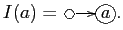There is always a map of graphs $\xi:I(a) \to G$. For instance for the input tree in the example we just discussed, $\xi$ is the map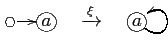Consequently if $(G,{ P})$ is a network and $I(a)$ is an input tree of a node of $G$, then $(I(a), { P}\circ \xi)$ is also a network. This allows us to talk about the phase space ${\mathbb{P}} I(a)$ of an input tree. In our running example,

${\mathbb{P}} I(a) = {\mathbb{R}}^2$

Given a network $(G,{ P})$, there is a vector space ${Ctrl}({\mathbb{P}} I(a)\to {\mathbb{P}} a)$ of open systems to every node $a$ of $G$. (THIS SENTENCE IS UNGRAMMATICAL AND THE CONCEPT OF vector space of open systems DOESN’T SOUND RIGHT - PROBABLY SOME TYPOS HERE. FURTHERMORE THE NOTATION $Ctrl$ WAS NEVER DEFINED - PERHAPS IT’S PART OF THE UNDEFINED CONCEPT OF control system???) In our running example the vector space associated to the one node $a$ of $(G',{ P}')$ is

${Ctrl}({\mathbb{R}}^2, {\mathbb{R}}) \simeq C^\infty({\mathbb{R}}^2, {\mathbb{R}})$

In the same example the network $(G,{ P})$ has three nodes and we associate the same vector space $C^\infty({\mathbb{R}}^2, {\mathbb{R}})$ to each one of them.

We then construct an interconnection map

$\displaystyle{ {{I}}: \bigsqcap_{a\in G_0} {Ctrl}({\mathbb{P}} I(a)\to {\mathbb{P}} a) \to \Gamma (T{\mathbb{P}}(G, { P})) }$

from the product of spaces of all control systems to the space of vector fields

$\Gamma (T{\mathbb{P}} (G, { P}))$

on the total phase space of the network. (We use the standard notation to denote the tangent bundle of a region $R$ by $TR$ and the space of vector fields on $R$ by $\Gamma (TR)$). In our running example the interconnection map for the network $(G',{ P}')$ is the map

$\displaystyle{ {{I}}: C^\infty({\mathbb{R}}^2, {\mathbb{R}}) \to C^\infty({\mathbb{R}}, {\mathbb{R}}), \quad f(x,u) \mapsto f(x,x). }$

The interconnection map for the network $(G,{ P})$ is the map

$\displaystyle{ {{I}}: C^\infty({\mathbb{R}}^2, {\mathbb{R}})^3 \to C^\infty({\mathbb{R}}^3, {\mathbb{R}}^3)}$

given by

$\displaystyle{ ({{I}}(f_1,f_2, f_3))\,(x_1,x_2, x_3) = (f_1(x_1,x_2), f_2(x_2,x_1), f_3(x_3,x_2)). }$

To summarize: a dynamical system on a network of regions is the data $(G, { P}, (w_a)_{a\in G_0} )$ where $G=\{G_1\rightrightarrows G_0\}$ is a directed graph, ${ P}:{ P}:G_0\to {{Region}}$ is a phase space function and $(w_a)_{a\in G_0}$ is a collection of open systems compatible with the graph and the phase space function. The corresponding vector field on the total space of the network is obtained by interconnecting the open systems.

Dynamical systems on networks can be turned into a category. Carrying this out gives us a way to associate maps of dynamical systems to combinatorial data.

The first step is to form the category of networks of regions, which we call ${{Graph}}/{{Region}}.$ In this category, by definition, a morphism from a network $(G,{ P})$ to a network $(G', { P}')$ is a map of directed graphs $\varphi:G\to G'$ which is compatible with the phase space functions:

$\displaystyle{ { P}'\circ \varphi = { P}. }$

Using the universal properties of products it is easy to show that a map of networks $\varphi: (G,{ P})\to (G',{ P}')$ defines a map ${\mathbb{P}}\varphi$ of total phase spaces in the opposite direction:

$\displaystyle{ {\mathbb{P}} \varphi: {\mathbb{P}} G' \to {\mathbb{P}} G. }$

In the category theory language the total phase space assignment extends to a contravariant functor

$\displaystyle{ {\mathbb{P}}: {({{Graph}}/{{Region}})}^{op} \to {{Region}}. }$

We call this functor the total phase space functor. In our running example, the map

${\mathbb{P}}\varphi:{\mathbb{R}} = {\mathbb{P}}(G',{ P}') \to {\mathbb{R}}^3 = {\mathbb{P}} (G,{ P})$

is given by

$\displaystyle{ {\mathbb{P}} \varphi (x) = (x,x,x). }$

Continuous-time dynamical systems also form a category, which we denote by ${DS}$. The objects of this category are pairs consisting of a region and a vector field on the region. A morphism in this category is a smooth map of regions that intertwines the two vector fields. That is

$\mathrm{Hom}_{DS} ((M,X), (N,Y)) = \{f:M\to N \mid Df \circ X = Y\circ f\}$

for any pair of objects $(M,X), (N,Y)$ in ${DS}$. In general morphisms in this category are difficult to determine explicitly. For example if $(M, X) = ((a,b), \frac{d}{dt})$ then a morphism from $(M,X)$ to some dynamical system $(N,Y)$ is simply a piece of an integral curve of the vector field $Y$ defined on an interval $(a,b)$. And if $(M, X) = (S^1, \frac{d}{d\theta})$ is the constant vector field on the circle then a morphism from $(M,X)$ to $(N,Y)$ is a periodic orbit of $Y$. Proving that a given dynamical system has a periodic orbit is usually hard.

Consequently, given a map of networks

$\varphi:(G,{ P})\to (G',{ P}')$

and a collection of open systems

$\{w'_{a'}\}_{a'\in G'_0}$

on $(G',{ P}')$ we expect it to be very difficult if not impossible to find a collection of open systems $\{w_a\}_{a\in G_0}$ so that

$\displaystyle{ {\mathbb{P}} \varphi: ({\mathbb{P}} G', {{I}}' (w'))\to ({\mathbb{P}} G, {{I}} (w)) }$

is a map of dynamical systems.

It is therefore somewhat surprising that there is a class of maps of graphs for which the above problem has an easy solution. The graph maps of this class are known by several different names. Following Boldi and Vigna we refer to them as graph fibrations. Note that despite what the name suggests, graph fibrations in general are not required to be surjective on nodes or edges. For example the inclusion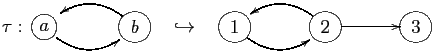is a graph fibration. We say that a map of networks

$\varphi:(G,{ P})\to (G',{ P}')$

is a fibration of networks if $\varphi:G\to G'$ is a graph fibration. With some work one can show that a fibration of networks induces a pullback map

$\displaystyle{ \varphi^*: \bigsqcap_{b\in G_0'} {Ctrl}({\mathbb{P}} I(b)\to {\mathbb{P} b) \to \bigsqcap_{a\in G_0} {Ctrl}({\mathbb{P}}} I(a)\to {\mathbb{P}} a) }$

on the sets of tuples of the associated open systems. The main result of Dynamics on networks of manifolds is a proof that for a fibration of networks $\varphi:(G,{ P})\to (G',{ P}')$ the maps $\varphi^*$, ${\mathbb{P}} \varphi$ and the two interconnection maps ${{I}}$ and ${{I}}'$ are compatible:

Theorem. Let $\varphi:(G,{ P})\to (G',{ P}')$ be a fibration of networks of manifolds. Then the pullback map

$\displaystyle{ \varphi^*: {Ctrl}(G',{ P}')\to {Ctrl}(G,{ P}) }$

is compatible with the interconnection maps

$\displaystyle{ {{I}}': {Ctrl}(G',{ P}')) \to \Gamma (T{\mathbb{P}} G') \quad and \quad {{I}}: ({Ctrl}(G,{ P})) \to \Gamma (T{\mathbb{P}} G). }$

Namely for any collection $w'\in {Ctrl}(G',{ P}')$ of open systems on the network $(G', { P}')$ the diagram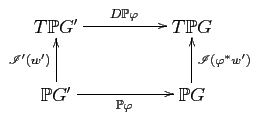commutes. In other words,

$\displaystyle{ {\mathbb{P}} \varphi: ({\mathbb{P}} (G',{ P}'), {{I}}' (w'))\to ({\mathbb{P}} (G, { P}), {{I}} (\varphi^* w')) }$

is a map of continuous time dynamical systems, a morphism in ${DS}$.

category: blog